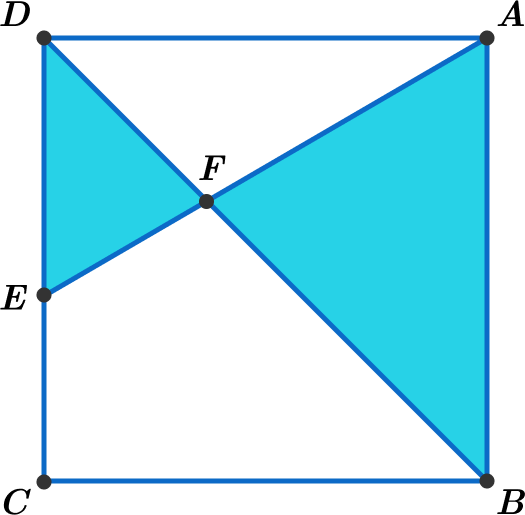# Insufficient Information in Geometry?

Geometry Level 3In the above diagram, $ABCD$ is a square and point $E$ lies on side $CD$.
Line segment $AE$ and diagonal $BD$ intersect at point $F$, such that $BF : FD = 4 : 3$.

If the combined area of the 2 blue triangles is $100 \text{ cm}^{2}$, find the area of the square in $\text{cm}^{2}$.


Note: The figure is not drawn to a scale

×